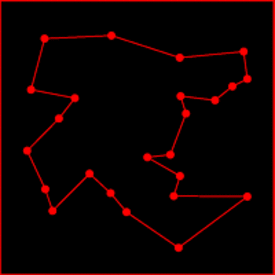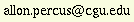### Discrete Mathematical Modeling

Fall 2019
Wednesdays 1-3:50pm
Burkle 24Instructor: Professor Allon Percus

Contact information: CGU Math South, tel. 909-607-0744Office hours: Wednesdays 10:30-11:30 at CGU Math South, or by appointment

Course description: Discrete mathematics deals with countable quantities. The techniques used for discrete models are often significantly different from those used for continuous models. This course explores some of the main techniques and problems that arise in discrete mathematical modeling. Topics include combinatorial analysis, Markov chains, graph theory, optimization, algorithmic behavior as well as current research topics. The goal is for students to acquire sufficient skills to solve real-world problems requiring discrete mathematical models.

Background and prerequisites: Prior exposure to combinatorics, probability and linear algebra is assumed. Students must have sufficient programming skills (in any language) to write a basic simulation code. Other background that is helpful but not required: an undergraduate-level course in discrete mathematics, and some exposure to analysis of algorithms.

Learning outcomes: It is expected that, by the end of this course, students will be able to:

• Understand and apply fundamental mathematical techniques needed for discrete modeling.
• Confidently solve real-world problems that involve discrete mathematical modeling.
• Develop valid algorithmic and computational approaches to implement discrete models.

Textbook: All required materials, including course notes, will be posted on Canvas under the “Files” section.

Tentative schedule:

• Sep 4: Combinatorial analysis. Product and sum rule; permutations and combinations; inclusion-exclusion; pigeonhole principle.
• Sep 11: Combinatorial analysis. Generating functions; algorithms and complexity.
Assignment #1 due
• Sep 18: Markov chains. Definitions; stationary distribution.
Assignment #2 due
• Sep 25: Markov chains. Sampling; card shuffling.
Assignment #3 due
• Oct 2: Markov chains. Markov-chain Monte Carlo; mixing time; spectral analysis.
Final project proposal due
• Oct 9: Markov chains. Strong stationary time.
• Oct 16: Graph theory. Connectivity; graph coloring.
Assignment #4 due
• Oct 23: Graph theory. Spanning trees; graph algorithms.
Assignment #5 due
• Oct 30: MIDTERM EXAM
• Nov 6: Combinatorial optimization. Minimum spanning trees; shortest path.
Assignment #6 due
• Nov 13: Combinatorial optimization. Network flows; heuristics; simulated annealing.
Assignment #7 due
• Nov 20: Random networks and algorithms. Random graph theory; zero-one laws; phase transitions.
• Nov 27: FINAL PROJECT WORKSHOP
• Dec 4: Random networks and algorithms. Random graph coloring; first moment method; algorithmic consequences.
• Dec 11: FINAL PROJECT PRESENTATIONS

Assignments and grading: Homework assignments will be posted on Canvas. All completed assignments must be submitted electronically as a pdf file, either by e-mail to the instructor or uploaded to Canvas. You may submit a scanned hardcopy, as long as it is in pdf format. I will also post the LaTeX source code along with each homework assignment; those of who choose to typeset your homework submissions in LaTeX may find this helpful. No late homework will be accepted, since solutions will be posted the morning after the due date. Graded homework will normally be returned, with feedback, one week after submission.

There will be an in-class midterm exam and a final project. Grades will be calculated as follows:

• 35% Homework assignments (lowest homework grade will be dropped)
• 30% Midterm exam
• 30% Final project
• 5% Class participation

You may work together on homework assignments. However, all your answers must be explained in your own words. Your homework submission must also state the names of all those with whom you collaborated. Please familiarize yourselves with CGU’s academic honesty policies.